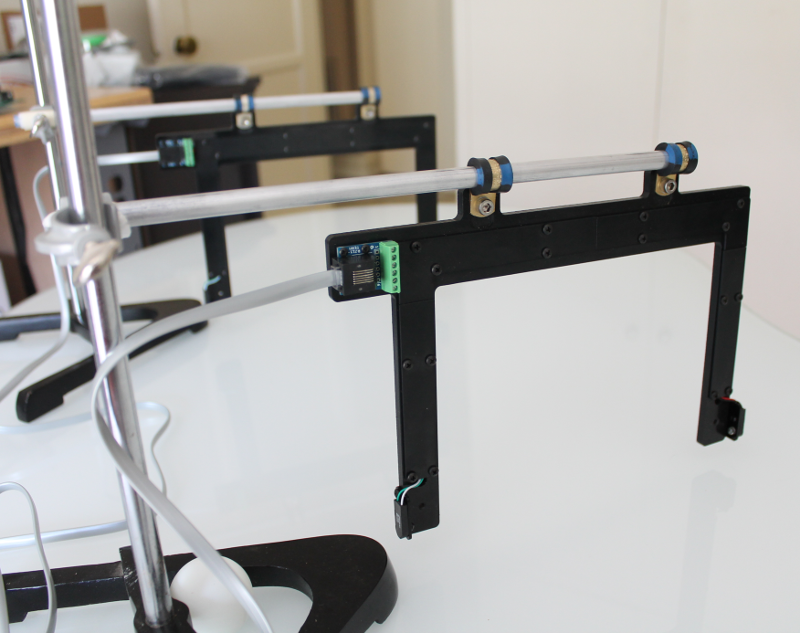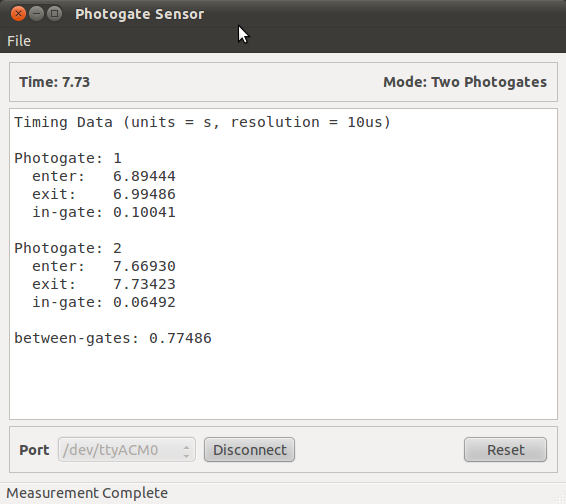# Inclined plane - rolling ball¶

The equation for the acceleration of the center of mass of a rolling sphere on an inclined plane  is:

$a_{\mathrm{cm}} = \frac{ g \sin(\theta) }{ \left( 1 + \frac{I}{M R^2} \right)}$

where $$a_{\mathrm{cm}}$$ is the acceleration of the center of mass, $$g$$ is the acceleration due to gravity, $$\theta$$ is the angle of incline, $$I$$ is the moment of inertia, $$M$$ is the mass and $$R$$ is the radius.

The moment of inertia, $$I$$ for a hollow sphere  is given by

$I = \frac{2 M R^2}{3}.$

Using this we can simplify the equation for the center mass as follows

$a_{\mathrm{cm}} = \frac{3}{5} g \sin(\theta).$

Solving for $$\theta$$ gives

$\theta = \sin^{-1} \left( \frac{5 a_\mathrm{cm} }{3 g} \right)$

## 1) Setting up the photogates¶

Place the two photogates on separate stands  on an inclined surface. in this case there were on a smooth glass table top set at a 2.5 degree slope.## 2) Software¶

Set-up the software as described in the previous section Using the photogate software.

## 3) Sample data¶

In the example shown below, we used a ping-pong ball (width = 0.040m) passing through the two photogates on a table with a slope of 2.5 degree. Place the ball on the table above photogate 1 and release so it rolls down the inclined table between the two photogates. Save data for analysis.## 4) Data analysis¶

• As in the previous experiment, use data from both photogates to calculate the acceleration, $$a_\mathrm{cm}$$ of the ball:

• Velocity in Gate 1 = 0.398 $$\mathrm{m}/\mathrm{s}$$
• Velocity in Gate 2 = 0.616 $$\mathrm{m}/\mathrm{s}$$
• $$a_\mathrm{cm}$$ = 0.281 $$\mathrm{m}/\mathrm{s}^2$$
• From equation, angle, $$\theta$$, is given by

$\theta \approx \sin^{-1} \left( \frac{5 \times 0.281}{3 \times 9.81} \right) \approx 2.73^{\circ}$

Footnotes

  http://iweb.tntech.edu/murdock/books/v2chap2.pdf. Example 5 on page 42.
  Additional equipment from Carolina Biologicals. Support Stand $15.50, Part # 707146 and Clamp Holder,$9.90, Part # 707310